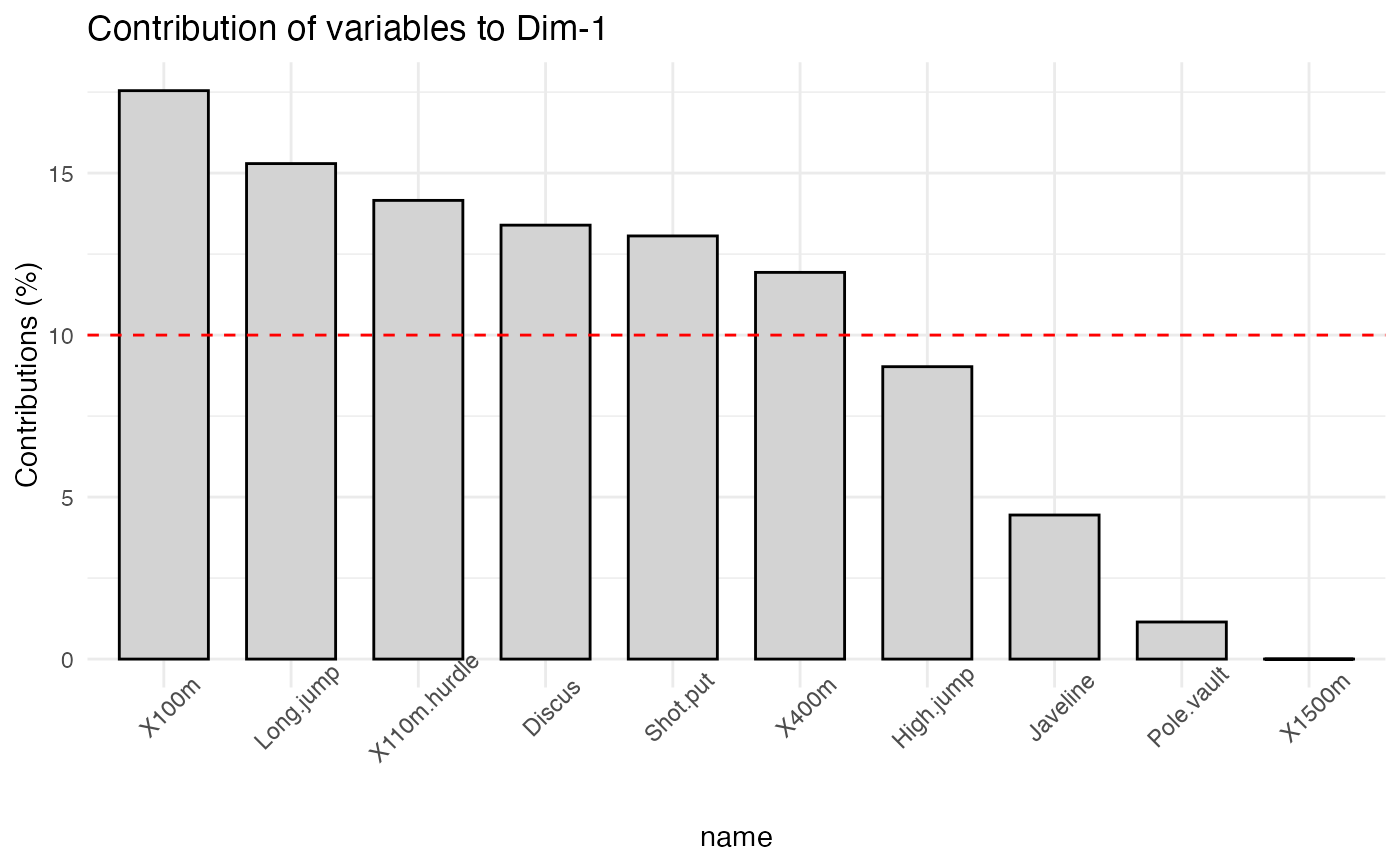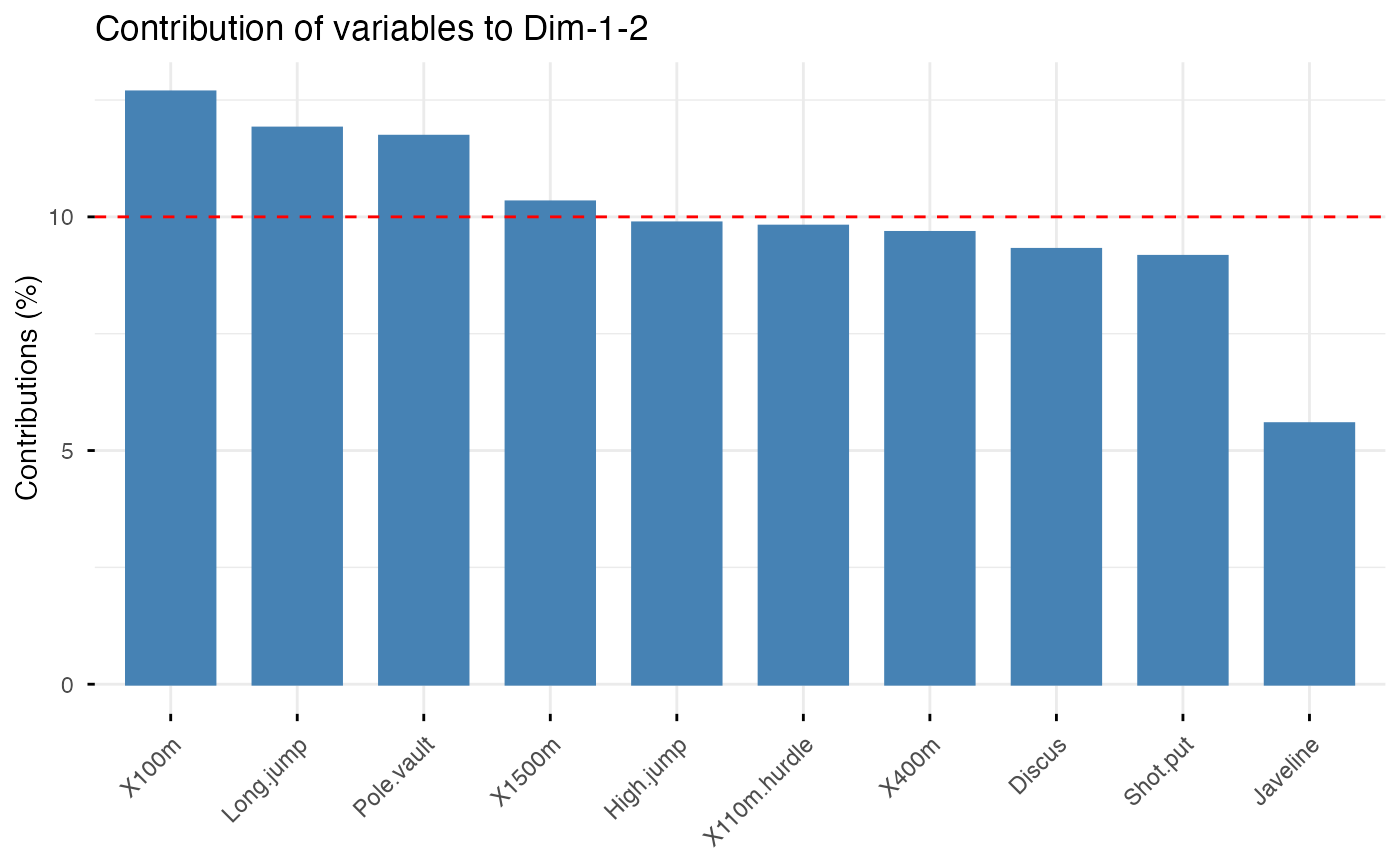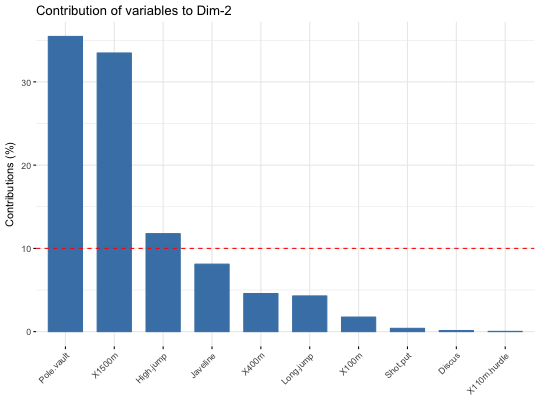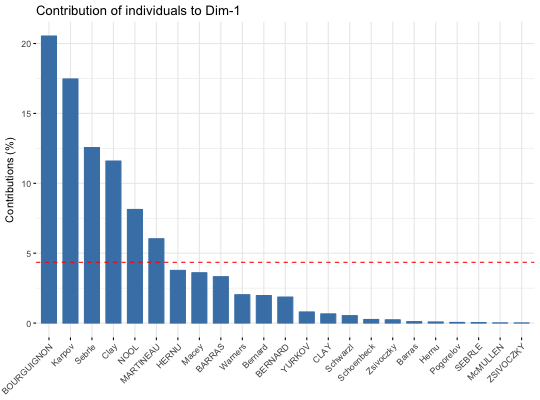This function can be used to visualize the contribution of rows/columns from the results of Principal Component Analysis (PCA), Correspondence Analysis (CA), Multiple Correspondence Analysis (MCA), Factor Analysis of Mixed Data (FAMD), and Multiple Factor Analysis (MFA) functions.

fviz_contrib(X, choice = c("row", "col", "var", "ind", "quanti.var",
"quali.var", "group", "partial.axes"), axes = 1, fill = "steelblue",
color = "steelblue", sort.val = c("desc", "asc", "none"), top = Inf,
xtickslab.rt = 45, ggtheme = theme_minimal(), ...)

fviz_pca_contrib(X, choice = c("var", "ind"), axes = 1,
fill = "steelblue", color = "steelblue", sortcontrib = c("desc", "asc",
"none"), top = Inf, ...)

## Arguments

X
an object of class PCA, CA, MCA, FAMD, MFA and HMFA [FactoMineR]; prcomp and princomp [stats]; dudi, pca, coa and acm [ade4]; ca [ca package].
choice
allowed values are "row" and "col" for CA; "var" and "ind" for PCA or MCA; "var", "ind", "quanti.var", "quali.var" and "group" for FAMD, MFA and HMFA.
axes
a numeric vector specifying the dimension(s) of interest.
fill
a fill color for the bar plot.
color
an outline color for the bar plot.
sort.val
a string specifying whether the value should be sorted. Allowed values are "none" (no sorting), "asc" (for ascending) or "desc" (for descending).
top
a numeric value specifying the number of top elements to be shown.
xtickslab.rt
Same as x.text.angle and y.text.angle, respectively. Will be deprecated in the near future.
ggtheme
function, ggplot2 theme name. Default value is theme_pubr(). Allowed values include ggplot2 official themes: theme_gray(), theme_bw(), theme_minimal(), theme_classic(), theme_void(), ....
...
other arguments to be passed to the function ggpar.
sortcontrib
see the argument sort.val

a ggplot2 plot

## Details

The function fviz_contrib() creates a barplot of row/column contributions. A reference dashed line is also shown on the barplot. This reference line corresponds to the expected value if the contribution where uniform. For a given dimension, any row/column with a contribution above the reference line could be considered as important in contributing to the dimension.

## Functions

• fviz_pca_contrib: deprecated function. Use fviz_contrib()

http://www.sthda.com/english/

## Examples

# Principal component analysis
# ++++++++++++++++++++++++++
data(decathlon2)
decathlon2.active <- decathlon2[1:23, 1:10]
res.pca <- prcomp(decathlon2.active,  scale = TRUE)

# variable contributions on axis 1
fviz_contrib(res.pca, choice="var", axes = 1, top = 10 )# Change theme and color
fviz_contrib(res.pca, choice="var", axes = 1,
fill = "lightgray", color = "black") +
theme_minimal() +
theme(axis.text.x = element_text(angle=45))# Variable contributions on axis 2
fviz_contrib(res.pca, choice="var", axes = 2)# Variable contributions on axes 1 + 2
fviz_contrib(res.pca, choice="var", axes = 1:2)# Contributions of individuals on axis 1
fviz_contrib(res.pca, choice="ind", axes = 1)## Not run: ------------------------------------
# # Correspondence Analysis
# # ++++++++++++++++++++++++++
# # Install and load FactoMineR to compute CA
# # install.packages("FactoMineR")
# library("FactoMineR")
# res.ca <- CA(housetasks, graph = FALSE)
#
# # Visualize row contributions on axes 1
# fviz_contrib(res.ca, choice ="row", axes = 1)
# # Visualize column contributions on axes 1
# fviz_contrib(res.ca, choice ="col", axes = 1)
#
# # Multiple Correspondence Analysis
# # +++++++++++++++++++++++++++++++++
# library(FactoMineR)
# data(poison)
# res.mca <- MCA(poison, quanti.sup = 1:2,
#               quali.sup = 3:4, graph=FALSE)
#
# # Visualize individual contributions on axes 1
# fviz_contrib(res.mca, choice ="ind", axes = 1)
# # Visualize variable categorie contributions on axes 1
# fviz_contrib(res.mca, choice ="var", axes = 1)
#
# # Multiple Factor Analysis
# # ++++++++++++++++++++++++
# library(FactoMineR)
# data(poison)
# res.mfa <- MFA(poison, group=c(2,2,5,6), type=c("s","n","n","n"),
#                name.group=c("desc","desc2","symptom","eat"),
#                num.group.sup=1:2, graph=FALSE)
#
# # Visualize individual contributions on axes 1
# fviz_contrib(res.mfa, choice ="ind", axes = 1, top = 20)
# # Visualize catecorical variable categorie contributions on axes 1
# fviz_contrib(res.mfa, choice ="quali.var", axes = 1)
## ---------------------------------------------